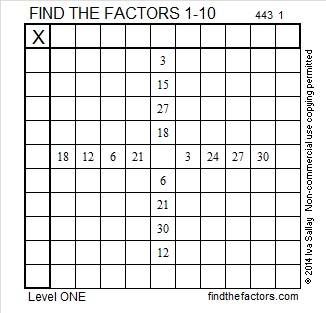# 276 and Level 1

• 276 is a composite number.
• Prime factorization: 276 = 2 x 2 x 3 x 23, which can be written 276 = (2^2) x 3 x 23
• The exponents in the prime factorization are 2, 1 and 1. Adding one to each and multiplying we get (2 + 1)(1 + 1)(1 + 1) = 3 x 2 x 2 = 12. Therefore 276 has 12 factors.
• Factors of 276: 1, 2, 3, 4, 6, 12, 23, 46, 69, 92, 138, 276
• Factor pairs: 276 = 1 x 276, 2 x 138, 3 x 92, 4 x 69, 6 x 46, or 12 x 23
• Taking the factor pair with the largest square number factor, we get √276 = (√4)(√69) = 2√69 ≈ 16.613I’m excited that resourceaholic included a FIND THE FACTORS puzzle in a gems post. Check it out! There are several other interesting resources there, too.Print the puzzles or type the factors on this excel file: 10 Factors 2014-10-27This site uses Akismet to reduce spam. Learn how your comment data is processed.